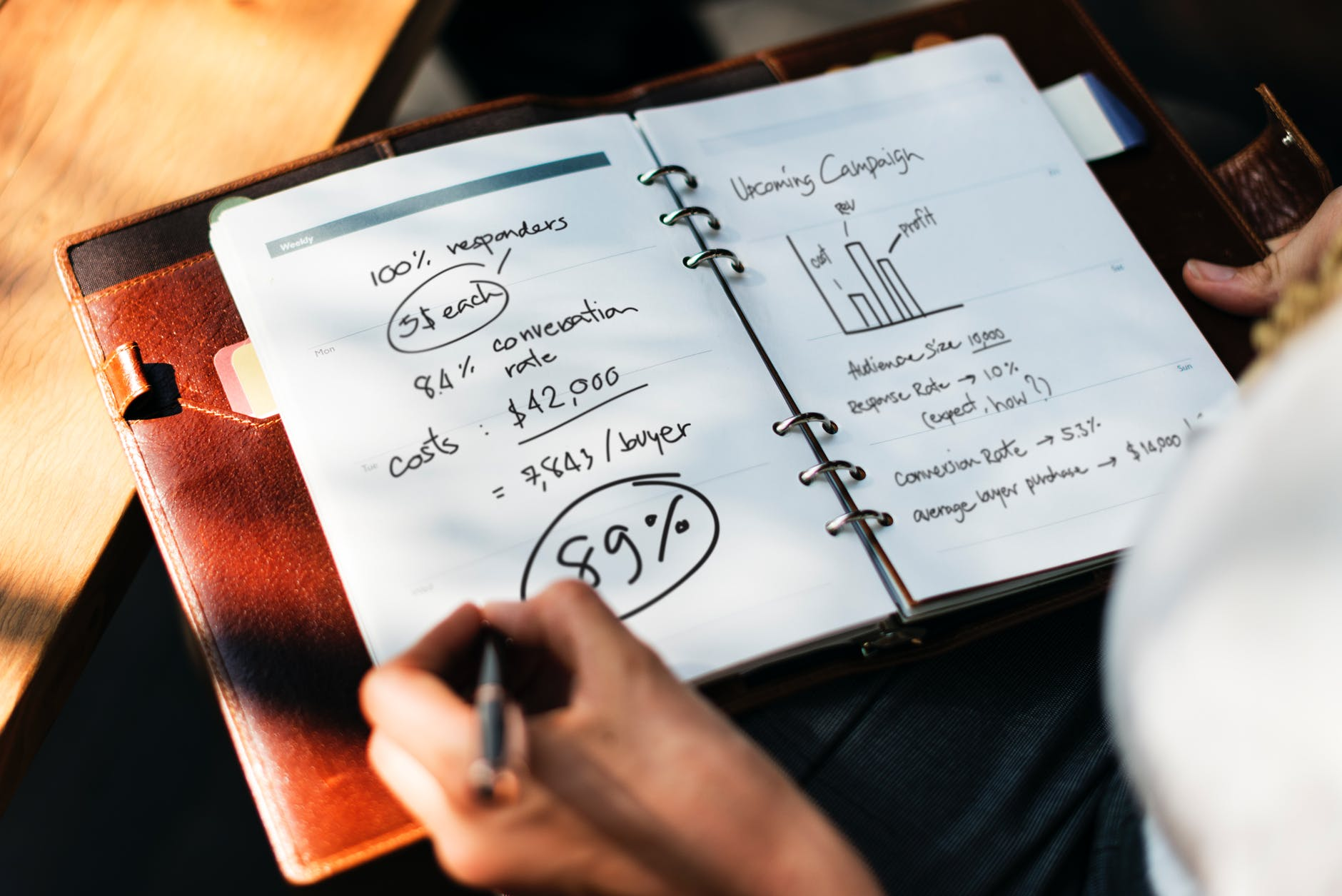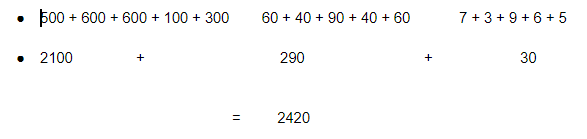# Everything you should know about Mental Math

## What is mental math?

We will jump right to definition but first, let me just ask, what’s 3 + 3 ?

Almost all of you have given the right answer  and most probably within 2 seconds without even touching a pen or paper.

The real question is how?

This is because our brain is already been trained to do simple calculations like these. It have already achieved a state where we no longer required special tools to do basic arithmetic calculations. This ability to mathematical calculations quick and without any help is called  Mental Math.

Mental Math is our ability to do mathematical calculations in our mind without help of any device, such as calculator and mobile phones etc. The more we develop mental math skills, faster our brain will have the  computational power. The level of mental math depends upon the amount of practice and the environment in which we practice our mathematical skills.

Also, the level of education plays an important role.  Obviously, there are some exceptions that may contradict this.

How  effective mental math is for dyscalculia?

In order to answer this question,  first we need to consider the fact that dyscalculia is a disorder whereas Mental Math is  more of a habit. Dyscalculia is an issue that needs to be attended first before jumping into developing our mental math skills. I mean you can’t teach a baby to run while he is struggling to take his first few steps.

As the child grows and begin developing the number sense , Mental math become equally important in his/her routine. We have already discussed in our previous blog post on dyscalculia that other than understanding numbers , Dyscalculia is also marked by visual difficulties such as  processing and distinguishing visuals as well as pattern recognition and at the same time, Mental  math acts as a visualisation workshop for our brain. Every time an arithmetic question is thrown to us, our brain starts looking for the raw material – which are the numbers ,Tools -which are the operators and finally, it starts the operation to make our final product – that is the answer. In general sequence, we take first number and then the second number, then you look for all operators  and then finally do the operation. For an individual suffering from dyscalculia, this effectively improve the visual representation of numbers.

## How to practice mental math?

Broadly, there are two ways in which you can effectively  practice and improve Mental math.

Theoretical approach  In this kind of approach, initially we learn  mathematical concepts by writing on paper and  and then practice the concepts in other ways orally. The idea behind this is that to have a sound knowledge of every aspect of solving a problem so that our brain gets comfortable solving it without the help of any device. However, there is no single way to follow this approach. Different problems have different ways. It totally depends upon the comfortability of child. We would explain by taking one of the most commonly used technique.

Let us take example of cumbersome sum of 5 numbers, say 567, 643, 699, 146, 365 .

First we will going to solve it as we normally do (on paper) . Take a stopwatch and note down the timings. Do follow the steps accordingly.

1. Start the timer. Solve

567  +  643  +  699  +  146  +  365

Got the answer? Note down the time

2. Now try to do it in your mind and note down the timing.

Next, we will write down a new way of solving it. Let’s write the sum like thisNow try doing it in your mind and note down the timing.

You  will notice that although  it took more time to do the calculation on paper with second method, it was way easier and took less time when you did it in mind. Still it is unfair that we already know the answer while solving with the second method. Hence, it is recommended that you use this method first and then move to the normal one. Also try comparing the results by using different numbers. Best way to use this method used is to create a pre-made template in your mind.

A general picture of the template would be like thisMemorize this  template and use it repeatedly for  lengthy calculations. Our brain memorize way better when information  is sequentially provided in small pieces than provided randomly. This is the main reason behind the structural aspects of this template. It took less effort for our brain to process the numerical information this way.

Physical approach

Physical approach involves our interaction with tangible real life objects. You come across several examples in daily life where you can improve your mental math skills. The main idea behind this approach is to train our brain on effectively picturing the scenario whenever a related arithmetic question is asked.

Let’s dig a little deeper by using some real life examples :

Staircase

Steps in staircase can greatly help in practicing number sequence and series. It improves counting for dyscalculics. It’s mostly helpful for training our brain for faster addition and low level multiplication.

For addition, put numbers on steps with temporary marker. Stand on number 1. Now start climbing the step number of the addition result. Let’s add 1 +5, which is 6. Start taking steps to reach 6. Do not jump. Take steps one by one.Corners around the world

Squares and rectangles are in lot of numbers around us. May it doors, tiles, tables, room wall or egg tray. Imagine dividing the shape in horizontal as well as vertical lines. Start from 2 horizontal and 1 vertical line. Count the number of parts. Multiplication is effectively improved through this. Now, step by step, start increasing the number of lines. Do it for both the lines individually. Tiled floor room is the best option if you have trouble imagining the lines.

Abacus

Abacus  is  a manual aid to calculating that consists of beads or disks that can be moved up and down on a series of sticks or strings within a usually wooden frame. It is consider one of the best way to develop number sense. Numbers are physically constructed and manipulated in Abacus. Practicing 10-15 minutes daily can effectively build your math calculative skills.